# Inclined plane

The body stays on an inclined plane and exerts a compressive force of 70N on it.
Find the angle between the inclined plane and the horizontal if a gravitational force of 100N acts on the body.

Correct result:

A =  44.427 °

#### Solution: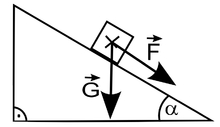We would be pleased if you find an error in the word problem, spelling mistakes, or inaccuracies and send it to us. Thank you!Tips to related online calculators

#### You need to know the following knowledge to solve this word math problem:

We encourage you to watch this tutorial video on this math problem:

## Next similar math problems:The regular quadrilateral pyramid has a base edge a = 1.56 dm and a height h = 2.05 dm. Calculate: a) the deviation angle of the sidewall plane from the base plane b) deviation angle of the side edge from the plane of the base
• Sphere in coneA sphere is inscribed in the cone (the intersection of their boundaries consists of a circle and one point). The ratio of the surface of the ball and the contents of the base is 4: 3. A plane passing through the axis of a cone cuts the cone in an isoscele
• Digging a pit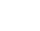The pit has the shape of a regular quadrilateral truncated pyramid. The edges of the bases are 14m and 10m long. The sidewalls form an angle of 135° with a smaller base. Determine how many m3 of soil were excavated when digging the pit?
• Maximum of volumeThe shell of the cone is formed by winding a circular section with a radius of 1. For what central angle of a given circular section will the volume of the resulting cone be maximum?
• Five circlesOn the line segment CD = 6 there are 5 circles with radius one at regular intervals. Find the lengths of the lines AD, AF, AG, BD, and CE
• Find the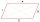Find the content of a regular 12 sided polygon, if its side a = 12 cm.
• Inscribed and described circle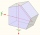Find the radii of a circle inscribed and circumscribed by a regular pentagon whose side measures 3 cm.
• Distance of pointsA regular quadrilateral pyramid ABCDV is given, in which edge AB = a = 4 cm and height v = 8 cm. Let S be the center of the CV. Find the distance of points A and S.
• Diagonal BDFind the length of the diagonal BD in a rectangular trapezoid ABCD with a right angle at vertex A when/AD / = 8,1 cm and the angle DBA is 42°
• ParallelogramFind the perimeter of the parallelogram, where base a = 8 cm, height v = 3 cm, and angle alpha = 35° is the magnitude of the angle at vertex A.
• Acute triangle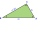In the acute triangle KLM, V is the intersection of its heights and X is the heel of height to the side KL. The axis of the angle XVL is parallel to the side LM and the angle MKL is 70°. What size are the KLM and KML angles?
• As shownAs shown, in △ ABC, ∠C = 90°, AD bisects ∠BAC, DE⊥AB to E, BE = 2, BC = 6, then the perimeter of △ BDE
• The staircaseThe staircase has a total height of 3.6 m and forms an angle of 26° with the horizontal. Calculate the length of the whole staircase.
• Powerplant chimneyFrom the window of the building at a height of 7.5 m, the top of the factory chimney can be seen at an altitude angle of 76° 30 ′. The base of the chimney can be seen from the same place at a depth angle of 5° 50 ′. How tall is the chimney?
• SailboatThe 20 m long sailboat has an 8 m high mast in the middle of the deck. The top of the mast is fixed to the bow and stern with a steel cable. Determine how much cable is needed to secure the mast and what angle the cable will make with the ship's deck.Calculate the content of a regular 15-sides polygon inscribed in a circle with radius r = 4. Express the result to two decimal places.Calculate the size of the smaller of the angles determined by lines A1 A4 and A2 A10 in the regular dodecagon A1A2A3. .. A12. Express the result in degrees.